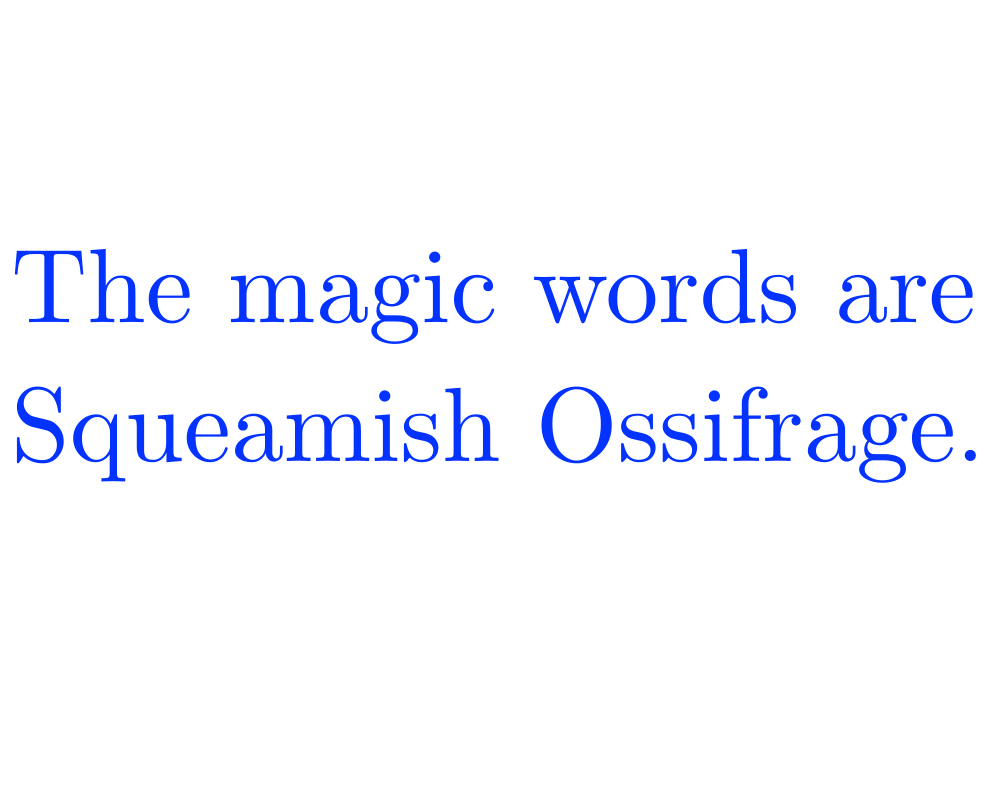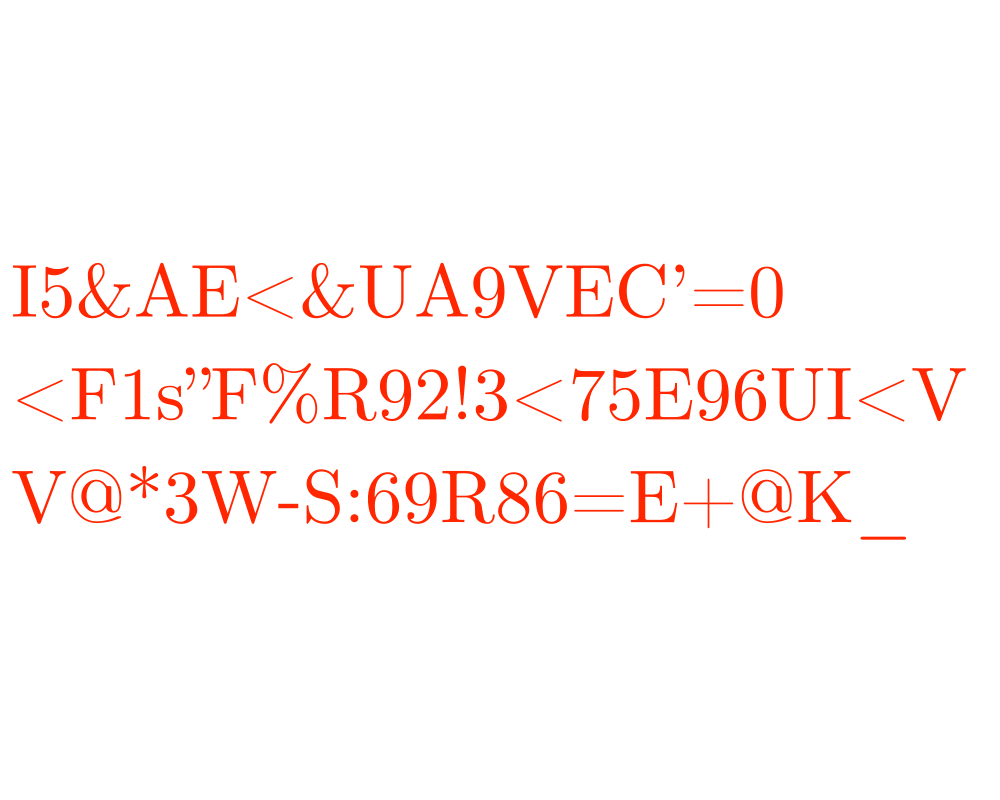# CryptographyInputOutput

Input Description: A plaintext message $$T$$ or encrypted text $$E$$, and a key $$k$$.
Problem: Encode $$T$$ using $$k$$ giving $$E$$, or decode $$E$$ using $$k$$ back to $$T$$.

Excerpt from The Algorithm Design Manual: Cryptography has grown substantially in importance in recent years, as computer networks have made confidential documents more vulnerable to prying eyes. Cryptography is a way to increase security by making messages difficult to read if they fall into the wrong hands. Although the discipline of cryptography is at least two thousand years old, its algorithmic and mathematical foundations have recently solidified to the point where there can now be talk of provably secure cryptosystems.

The key issue in selecting a cryptosystem is identifying your paranoia level, i.e. deciding how much security you need. Who are you trying to stop from reading your stuff: your grandmother, local thieves, the Mafia, or the NSA? If you can use an accepted implementation of RSA, such as PGP discussed below, you should feel safe against just about anybody.

Go To Main Page